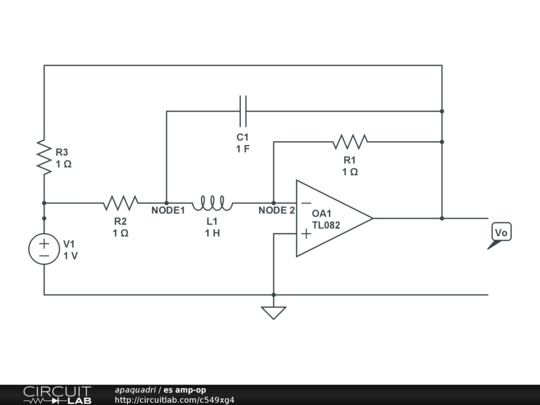# Calculate transfer function with Sine generator

Given this circuit I would like to know how can I find it's transfer function.

A method I've considered was to use Node Equations on nodes 1 and 2 , and I have a few things that I don't find clear :

1. why do I have to introduce an additional equation with $V2=0$ ?
2. Once I find the transfer function in form of $H(s) = {\dfrac{R1}{R2+Ls+CR1R2s+CLR2s^2}}$ I must calculate $Vu(t)$ with $V1=10+cos(t)$ , the solution states that $Vu(t)= H(0)*10 + Re[H(j)*e^{(jt)}*1]=-10-{\dfrac{1}{2}}{sin(t)}$ How does one achieve this result and first of all how does the $Re$ part of the transfer function is calculated ?

Thanks a lot for any insight provided.